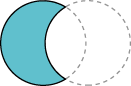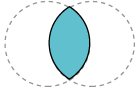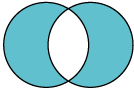# Constructing Complex Shapes from Geometry Primitives

Constructive area geometry (CAG) is the process of creating new geometric shapes by performing boolean operations on existing ones. In the Java 2D API the `Area` class implements the `Shape` interface and supports the following boolean operations.UnionSubtractionIntersectionExclusive-or (`XOR`)

## Example: Areas

In this example `Area` objects construct a pear shape from several ellipses.

Note:  If you don't see the applet running, you need to install at least the Java SE Development Kit (JDK) 7 release.

`Pear.java` contains the complete code for this applet.

The leaves are each created by performing an intersection on two overlapping circles.

```leaf = new Ellipse2D.Double();
...
leaf1 = new Area(leaf);
leaf2 = new Area(leaf);
...
leaf.setFrame(ew-16, eh-29, 15.0, 15.0);
leaf1 = new Area(leaf);
leaf.setFrame(ew-14, eh-47, 30.0, 30.0);
leaf2 = new Area(leaf);
leaf1.intersect(leaf2);
g2.fill(leaf1);
...
leaf.setFrame(ew+1, eh-29, 15.0, 15.0);
leaf1 = new Area(leaf);
leaf2.intersect(leaf1);
g2.fill(leaf2);
```

Overlapping circles are also used to construct the stem through a subtraction operation.

```stem = new Ellipse2D.Double();
...
stem.setFrame(ew, eh-42, 40.0, 40.0);
st1 = new Area(stem);
stem.setFrame(ew+3, eh-47, 50.0, 50.0);
st2 = new Area(stem);
st1.subtract(st2);
g2.fill(st1);
```

The body of the pear is constructed by performing a union operation on a circle and an oval.

```circle = new Ellipse2D.Double();
oval = new Ellipse2D.Double();
circ = new Area(circle);
ov = new Area(oval);
...
circle.setFrame(ew-25, eh, 50.0, 50.0);
oval.setFrame(ew-19, eh-20, 40.0, 70.0);
circ = new Area(circle);
ov = new Area(oval);